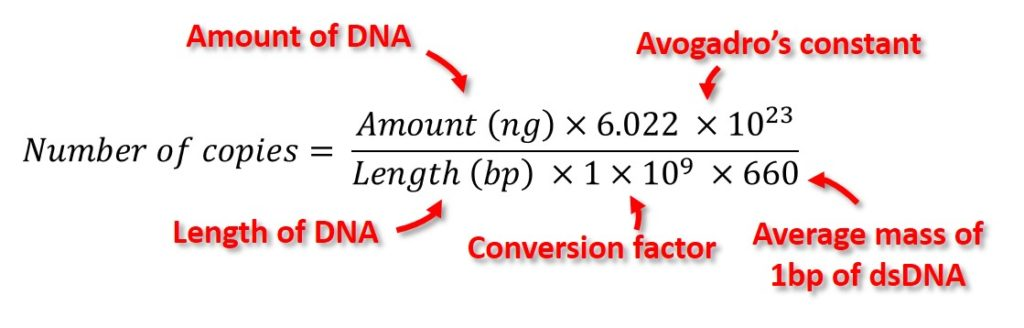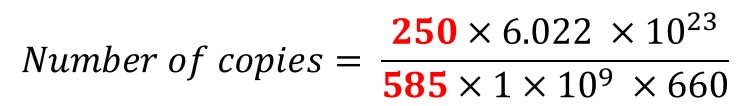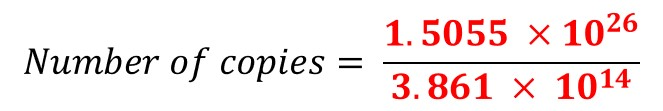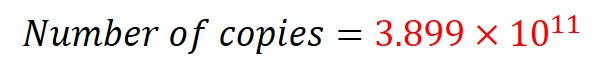# How To Calculate DNA Copy Number For qPCR

In this article, I will show you how to calculate the DNA copy number for qPCR, by using the known amount and length of DNA.

Calculating the DNA copy number is an important step in absolute qPCR since the number of copies are required during the creation of the standard curve.

## Mastering qPCR

Additional information on qPCR can be found in our Mastering qPCR course.
>>Use code 20QPCR to get 20% off<<

## How to calculate the number of copies of DNA

To estimate the DNA copy number, there are 2 pieces of information you need:

• Amount of DNA – The amount of DNA in the tube, measured in nanograms (ng), needs to be known.
• Length of DNA template – The number of base pairs (bp) of the DNA template is also required.

Once ready, this information can be entered into the equation below to calculate the number of copies.The other components of the equation are explained in more detail below.

• Avogadro’s constant – This number (6.022 x 1023) represents the number of molecules in 1 mole.
• Conversion factor – The conversion factor (1 x 109) is required to convert the value to ng.
• The average mass of 1 bp of dsDNA – The average mass of 1 bp of dsDNA is 660 g/mole. If the template was single-stranded, as opposed to double-stranded, this value should be changed to 330 g/mole.

## Make sure there is a single amplicon in the tube

If you are using the equation to calculate the number of copies of DNA for qPCR, ensure the tube contains only the amplicon you are interested in. If there are multiple amplicons, such as primer-dimer or unintended amplicons, then the resulting calculations will not be accurate.

Always run some product on an agarose gel electrophoresis, or double check the melt curve if using intercalating dyes, to confirm the presence of unintended amplicons.

## Example of using the equation

Calculate the number of copies of a DNA amplicon of 585 bp in length in a sample containing 250 ng.

1. Firstly, enter the known information into the equation.2. Next, do the maths. Start by performing the calculations on the top part of the equation, then the bottom half of the equation. You may need to use a scientific calculator for this, as the answers can be very large!3. Then, simply divide the top answer by the bottom answer.In the example above, therefore, there are 3.899 x 1011 copies of DNA in the tube.

## Use our DNA copy number online calculator

Are you still struggling with the maths? If so, I have also created an online calculator to help further.

Simply click here to go to the calculator. Enter the amount and length of the DNA, and select whether the template is single-or double-stranded DNA. Then the number of DNA copies will be calculated for you.

## The result is only an estimate

It is also important to note that the equation to calculate DNA copy numbers by using the amount and length of the template provides only an estimate. Simply, because the equation uses the average mass of 1 bp of dsDNA (i.e. the 660 g/mole in the equation), it does not account for the composition of the DNA template.

Each DNA template will be composed of different bases, each of which has a slightly different weight. So, for a more accurate calculation, the sequence of the DNA template should also be known.

However, if your purpose is to use the equation for qPCR, then it is usually sufficient.

#### 1 COMMENT

1.Dr Steven Bradburn, PhD